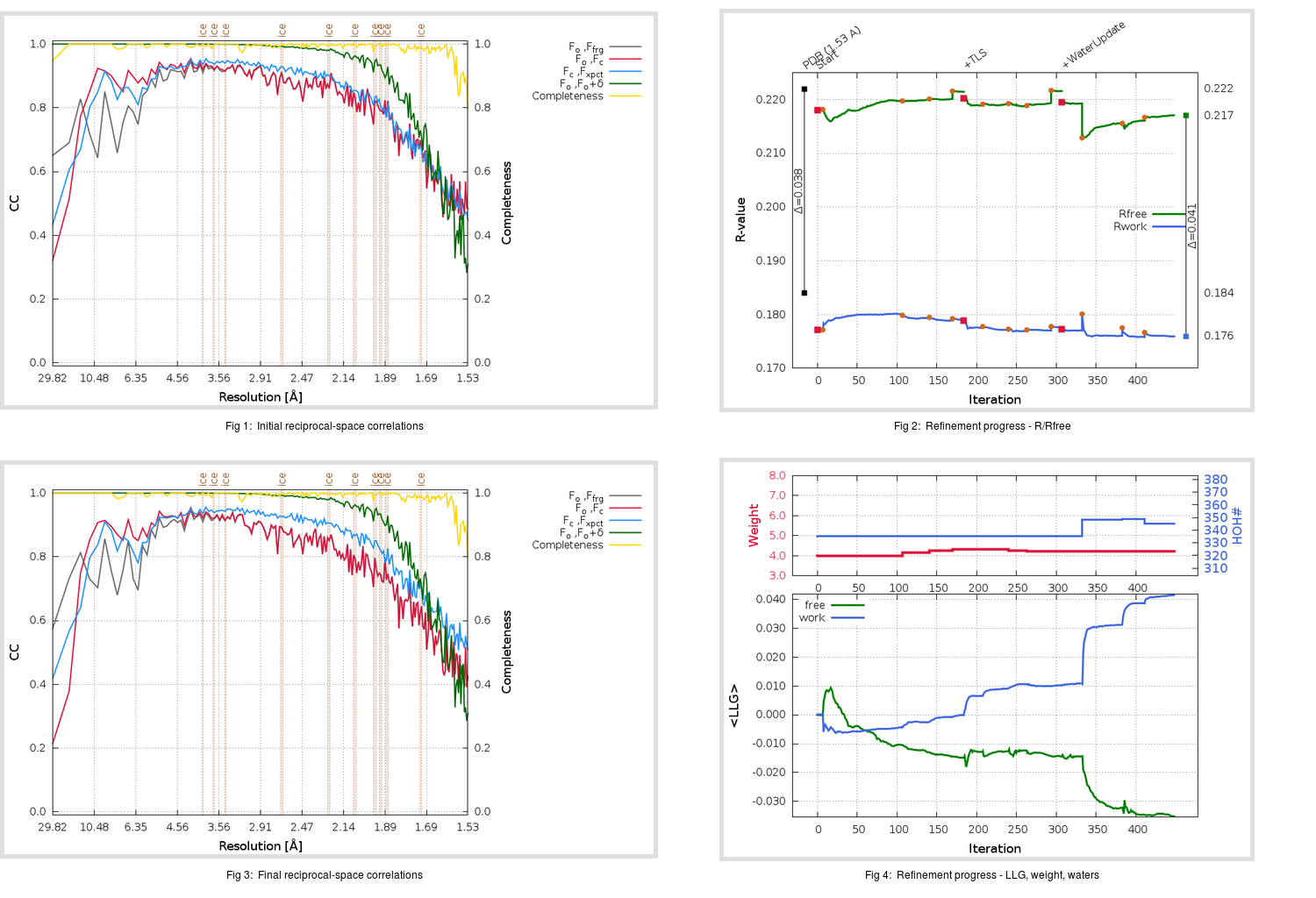Content:

```    Diffraction limits & principal axes of ellipsoid fitted to diffraction cut-off surface:
1.817         0.9845   0.0000  -0.1754       0.988 a* - 0.156 c*
1.501         0.0000   1.0000   0.0000       b*
1.614         0.1754   0.0000   0.9845       0.433 a* + 0.901 c*
```

## Deposited

` `
 Date deposited Date data collection Resolution R, Rfree 20200315 20200306 1.53 0.1820 0.2220

Molprobity (CCP4 7.0 version) summary:

```Ramachandran outliers =   0.33 %
favored =  98.01 %
Rotamer outliers      =   0.38 %
C-beta deviations     =     0
Clashscore            =   3.60
RMS(bonds)            =   0.0134
RMS(angles)           =   1.79
MolProbity score      =   1.15
Resolution            =   1.53
R-work                =   0.1820
R-free                =   0.2220
```

```Number of waters      =   335

<B> (all atoms) =   22.94 ( sd =    8.48 ) for       2722 non-hydrogen atoms
<B>   (protein) =   21.32 ( sd =    7.13 ) for       2370 non-hydrogen atoms
<B>     (water) =   33.68 ( sd =    8.92 ) for        335 non-hydrogen atoms
<B>    (others) =   35.32 ( sd =    6.00 ) for         17 non-hydrogen atoms

B min/max       (all non-hydrogen atoms) =   11.69 /   58.60
B min/max   (protein non-hydrogen atoms) =   11.69 /   58.60
B min/max     (water non-hydrogen atoms) =   12.13 /   56.65
B min/max     (other non-hydrogen atoms) =   25.49 /   48.34
```

## BUSTER (re-)refinement

` `

Molprobity (CCP4 7.0 version) summary:

```Ramachandran outliers =   0.33 %
favored =  97.68 %
Rotamer outliers      =   1.52 %
C-beta deviations     =     0
Clashscore            =   1.91
RMS(bonds)            =   0.0112
RMS(angles)           =   1.55
MolProbity score      =   1.16
Resolution            =   1.53
R-work                =   0.1760
R-free                =   0.2171
```

```Number of waters      =   345

<B> (all atoms) =   24.53 ( sd =    8.45 ) for       2732 non-hydrogen atoms
<B>   (protein) =   22.67 ( sd =    6.52 ) for       2370 non-hydrogen atoms
<B>     (water) =   36.64 ( sd =    9.68 ) for        345 non-hydrogen atoms
<B>    (others) =   34.67 ( sd =    5.62 ) for         17 non-hydrogen atoms

B min/max       (all non-hydrogen atoms) =   11.72 /   68.83
B min/max   (protein non-hydrogen atoms) =   13.57 /   56.94
B min/max     (water non-hydrogen atoms) =   11.72 /   68.83
B min/max     (other non-hydrogen atoms) =   25.58 /   41.40
```

Refinement progression:Results:

` `
 File Remark 5RF2_aB_refine.01_03_refine.pdb.gz exact refinement commands are in header 5RF2_aB_refine.01_03_refine.mtz.gz including original deposited data and several re-refinement map coefficients 5RF2_aB_refine.01_03_BUSTER_model.cif.gz including any non-standard compound restraints 5RF2_aB_refine.01_03_BUSTER_refln.cif.gz